library(OLStrajr)

## Introduction

The OLStrajr package in R, designed as an adaptation of the OLStraj SAS macro reported in Carrig, Wirth, and Curran (2004), facilitates the generation of individual level plots. These plots can depict linear, quadratic, or both ordinary least squares (OLS) estimated trajectories, superimposed on the original data.

In the following demonstration, we use the rats dataset (for details, see ?OLStrajr::rats), which originates from Rogosa & Saner (1995). We will explore how the ‘regtype’ parameter of OLStraj can be used to study linear, quadratic, or both OLS-estimated trajectories.

### Displaying the rats Dataset

data(rats)
print(rats)
#>    Rat t0 t1  t2  t3  t4   Z
#> 1    1 61 72 118 130 176 170
#> 2    2 65 85 129 148 174 194
#> 3    3 57 68 130 143 201 187
#> 4    4 46 74 116 124 157 156
#> 5    5 47 85 103 117 148 155
#> 6    6 43 58 109 133 152 150
#> 7    7 53 62  82 112 156 138
#> 8    8 72 96 117 129 154 154
#> 9    9 53 54  87 120 138 149
#> 10  10 72 98 114 144 177 167

### Plotting Linear Trajectories

# Run OLS traj
rats_lin <- OLStraj(data = rats,
idvarname = "Rat",
predvarname = "Week",
outvarname = "Weight",
varlist = c("t0", "t1", "t2", "t3", "t4"),
timepts = c(0, 1, 2, 3, 4),
regtype = "lin",
int_bins = 3,
lin_bins = 3)

# Show linear trajectories
rats_lin$individual_plots #>$ols 1
#> geom_smooth() using formula = 'y ~ x'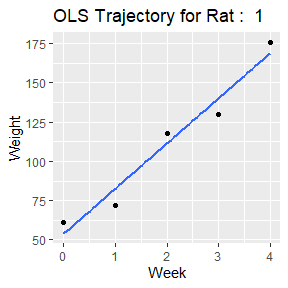#>
#> $ols 2 #> geom_smooth() using formula = 'y ~ x'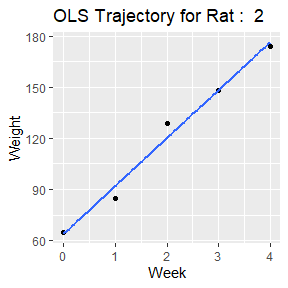#> #>$ols 3
#> geom_smooth() using formula = 'y ~ x'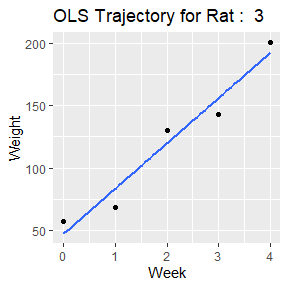#>
#> $ols 4 #> geom_smooth() using formula = 'y ~ x'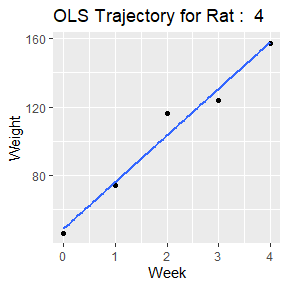#> #>$ols 5
#> geom_smooth() using formula = 'y ~ x'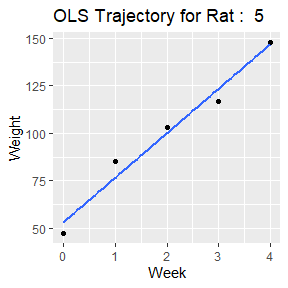#>
#> $ols 6 #> geom_smooth() using formula = 'y ~ x'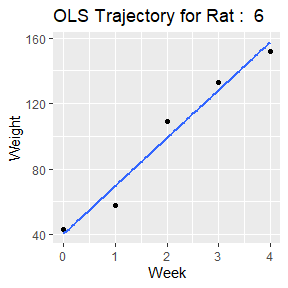#> #>$ols 7
#> geom_smooth() using formula = 'y ~ x'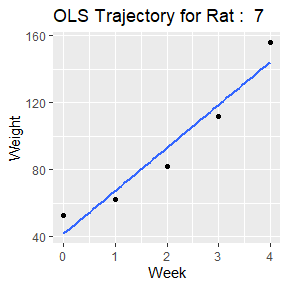#>
#> $ols 8 #> geom_smooth() using formula = 'y ~ x'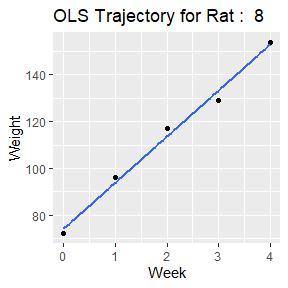#> #>$ols 9
#> geom_smooth() using formula = 'y ~ x'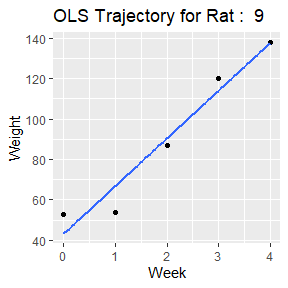#>
#> $ols 10 #> geom_smooth() using formula = 'y ~ x'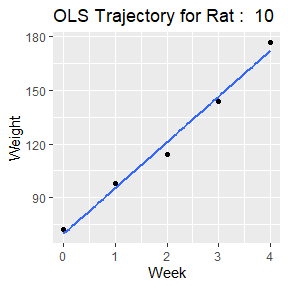Note: The cbc_lm vignette transforms the robbins data from wide to long format to run case-by-case regression. However, for models with a single independent variable, you can also obtain the cbc_lm output directly from the OLStraj results. print(rats_lin$models)
#> Call:
#> lm(formula = Weight ~ Week, data = mod_df)
#>
#> Mean Coefficients:
#> $(Intercept) #>  53.66 #> #>$Week
#>  26.76
#>
#>
#> Coefficients for each model:
#>
#> Model 1 coefficients:
#>    (Intercept)           Week
#>           53.8           28.8
#>
#> Model 2 coefficients:
#>    (Intercept)           Week
#>           64.0           28.1
#>
#> Model 3 coefficients:
#>    (Intercept)           Week
#>           47.2           36.3
#>
#> Model 4 coefficients:
#>    (Intercept)           Week
#>           49.0           27.2
#>
#> Model 5 coefficients:
#>    (Intercept)           Week
#>           53.2           23.4
#>
#> Model 6 coefficients:
#>    (Intercept)           Week
#>           40.4           29.3
#>
#> Model 7 coefficients:
#>    (Intercept)           Week
#>           41.8           25.6
#>
#> Model 8 coefficients:
#>    (Intercept)           Week
#>           74.2           19.7
#>
#> Model 9 coefficients:
#>    (Intercept)           Week
#>           43.2           23.6
#>
#> Model 10 coefficients:
#>    (Intercept)           Week
#>           69.8           25.6

OLStraj(data = rats,
idvarname = "Rat",
predvarname = "Week",
outvarname = "Weight",
varlist = c("t0", "t1", "t2", "t3", "t4"),
timepts = c(0, 1, 2, 3, 4),
int_bins = 3,
lin_bins = 3)$individual_plots #>$ols 1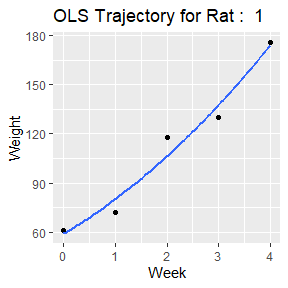#>
#> $ols 2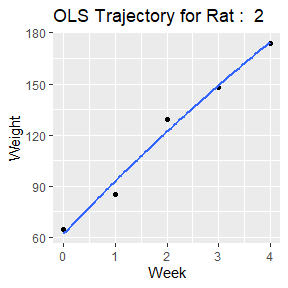#> #>$ols 3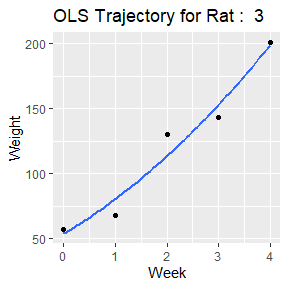#>
#> $ols 4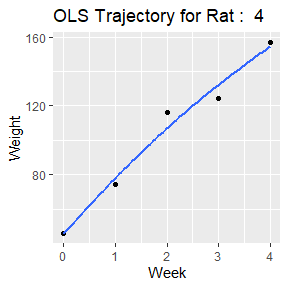#> #>$ols 5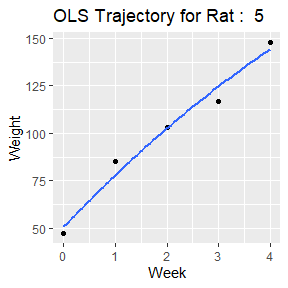#>
#> $ols 6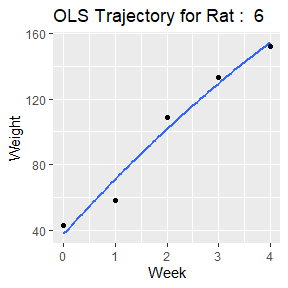#> #>$ols 7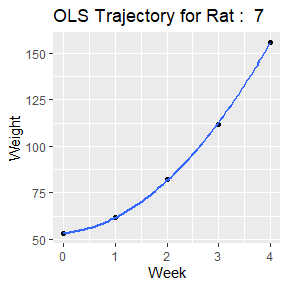#>
#> $ols 8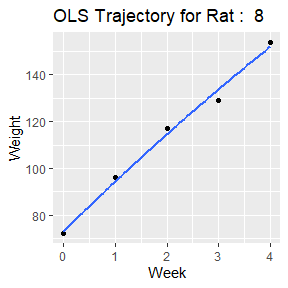#> #>$ols 9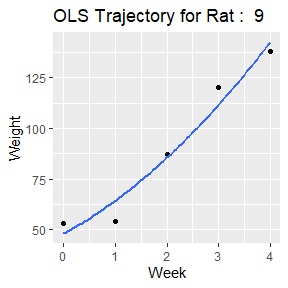#>
#> $ols 10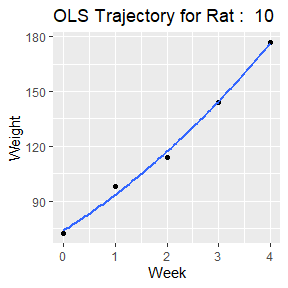### Plotting Linear & Quadratic Trajectories In the resulting plots, the quadratic trajectory is depicted by a dashed line while the linear trajectory is represented by a solid line. OLStraj(data = rats, idvarname = "Rat", predvarname = "Week", outvarname = "Weight", varlist = c("t0", "t1", "t2", "t3", "t4"), timepts = c(0, 1, 2, 3, 4), regtype = "both", int_bins = 3, lin_bins = 3)$individual_plots
#> $ols 1 #> geom_smooth() using formula = 'y ~ x'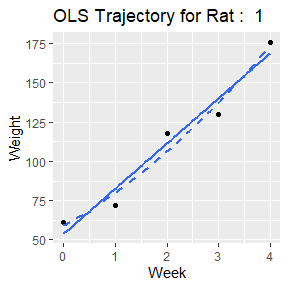#> #>$ols 2
#> geom_smooth() using formula = 'y ~ x'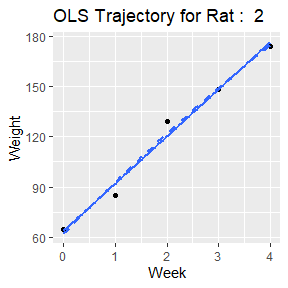#>
#> $ols 3 #> geom_smooth() using formula = 'y ~ x'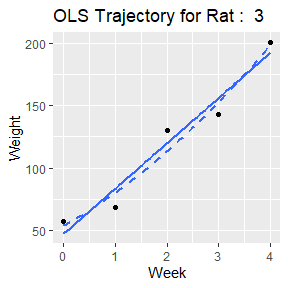#> #>$ols 4
#> geom_smooth() using formula = 'y ~ x'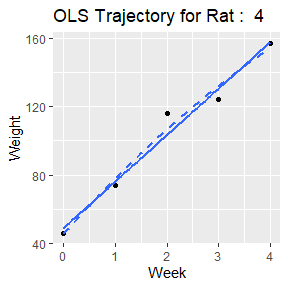#>
#> $ols 5 #> geom_smooth() using formula = 'y ~ x'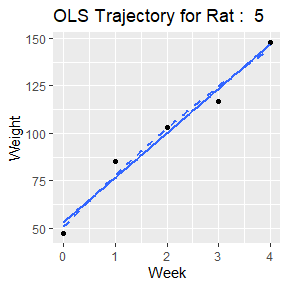#> #>$ols 6
#> geom_smooth() using formula = 'y ~ x'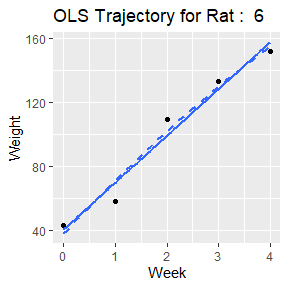#>
#> $ols 7 #> geom_smooth() using formula = 'y ~ x'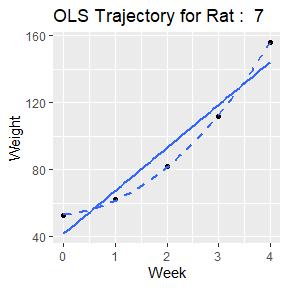#> #>$ols 8
#> geom_smooth() using formula = 'y ~ x'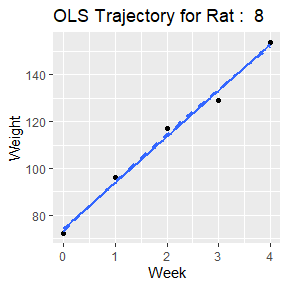#>
#> $ols 9 #> geom_smooth() using formula = 'y ~ x'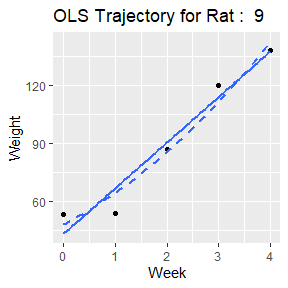#> #>$ols 10
#> geom_smooth() using formula = 'y ~ x'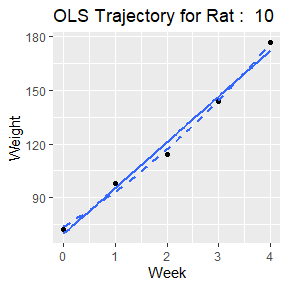The power of OLStrajr lies in its ability to quickly visualize the different approximations of the OLS trajectories. The package aids in the understanding of complex trends in the data and provides a solid base for further statistical analysis and interpretation.